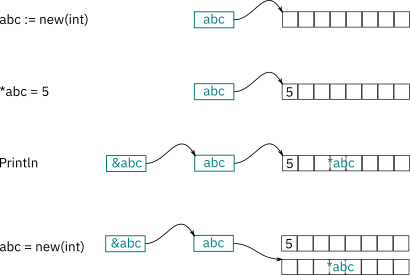# What does the memory change with pointers?

am confused how the addresses work though. When I run the app below I get this:

``````&abc                abc                   *abc
0xc42000c028 0xc420014080 5
0xc42000c028 0xc4200140a0 20
``````

So, &abc is the address of the data and *abc is the data pointed to by the address of abc.

Finally what is abc all by itself? Is that the address of where the abc variable is stored in memory. And why did it change when I created new(int). Why didn’t &abc change?

``````package main
import "fmt"
func main() {
abc := new(int)
*abc = 5
fmt.Println(&abc, abc, *abc)

// create new abc
abc = new(int)
*abc = 20
fmt.Println(&abc, abc, *abc)
}``````

`abc := new(int)` creates a `*int` and assigns it to `abc`.

`abc` itself has an address, you can ask for it by using `&`. So `&abc` is the memory location where the value (of type `*int`) is stored.

Next, as we already said, `abc` is a `*int`, reading a “pointer to an int". So again a memory address, which tells us where we find an `int`eger.

`*abc` then resolves (-> dereferences) the pointer to its actual value.

When you encounter the second `new(int)`, which uses the simple assignement operator (`=`), you reasign to the old `abc`, thats why `&abc` isn’t changing. Correct wording were “creating a new `*int`” since `abc` remains the same.

3 Likes

`abc := new(int)` allocates memory for an integer, and returns its address as a pointer to the memory.

Applying `&` to a variable returns a pointer to that variable, its address in memory. Applying `*` to a variable dereferences the pointer and returns the value.

So `abc` is a pointer to an int, `*abc` is the int itself, and `&abc` is the address of the pointer to an int – that is, a pointer to a pointer to an int.

If you replace abc with a different pointer to a different area of memory, the Go compiler will reuse the replaced variable, so the pointer to the pointer to the int will be the same, even though the pointer to the int is different.

Here’s a diagram.1 Like

This topic was automatically closed 90 days after the last reply. New replies are no longer allowed.